# Transform EEG data using current source density (CSD)#

This script shows an example of how to use CSD . CSD takes the spatial Laplacian of the sensor signal (derivative in both x and y). It does what a planar gradiometer does in MEG. Computing these spatial derivatives reduces point spread. CSD transformed data have a sharper or more distinct topography, reducing the negative impact of volume conduction.

```# Authors: Alex Rockhill <aprockhill@mailbox.org>
#
```
```import numpy as np
import matplotlib.pyplot as plt

import mne
from mne.datasets import sample

print(__doc__)

data_path = sample.data_path()
```

```meg_path = data_path / 'MEG' / 'sample'
raw = raw.pick_types(meg=False, eeg=True, eog=True, ecg=True, stim=True,
events = mne.find_events(raw)
raw.set_eeg_reference(projection=True).apply_proj()
```
```Opening raw data file /home/circleci/mne_data/MNE-sample-data/MEG/sample/sample_audvis_raw.fif...
Read a total of 3 projection items:
PCA-v1 (1 x 102)  idle
PCA-v2 (1 x 102)  idle
PCA-v3 (1 x 102)  idle
Range : 25800 ... 192599 =     42.956 ...   320.670 secs
Removing projector <Projection | PCA-v1, active : False, n_channels : 102>
Removing projector <Projection | PCA-v2, active : False, n_channels : 102>
Removing projector <Projection | PCA-v3, active : False, n_channels : 102>
Reading 0 ... 166799  =      0.000 ...   277.714 secs...
320 events found
Event IDs: [ 1  2  3  4  5 32]
EEG channel type selected for re-referencing
1 projection items deactivated
Average reference projection was added, but has not been applied yet. Use the apply_proj method to apply it.
Created an SSP operator (subspace dimension = 1)
1 projection items activated
SSP projectors applied...
```
Measurement date December 03, 2002 19:01:10 GMT MEG Unknown 146 points 9 Stimulus, 59 EEG, 1 EOG None EOG 061 Not available 600.61 Hz 0.10 Hz 172.18 Hz Average EEG reference : on sample_audvis_raw.fif 00:04:38 (HH:MM:SS)

Plot the raw data and CSD-transformed raw data:

```raw_csd = mne.preprocessing.compute_current_source_density(raw)
raw.plot()
raw_csd.plot()
```
•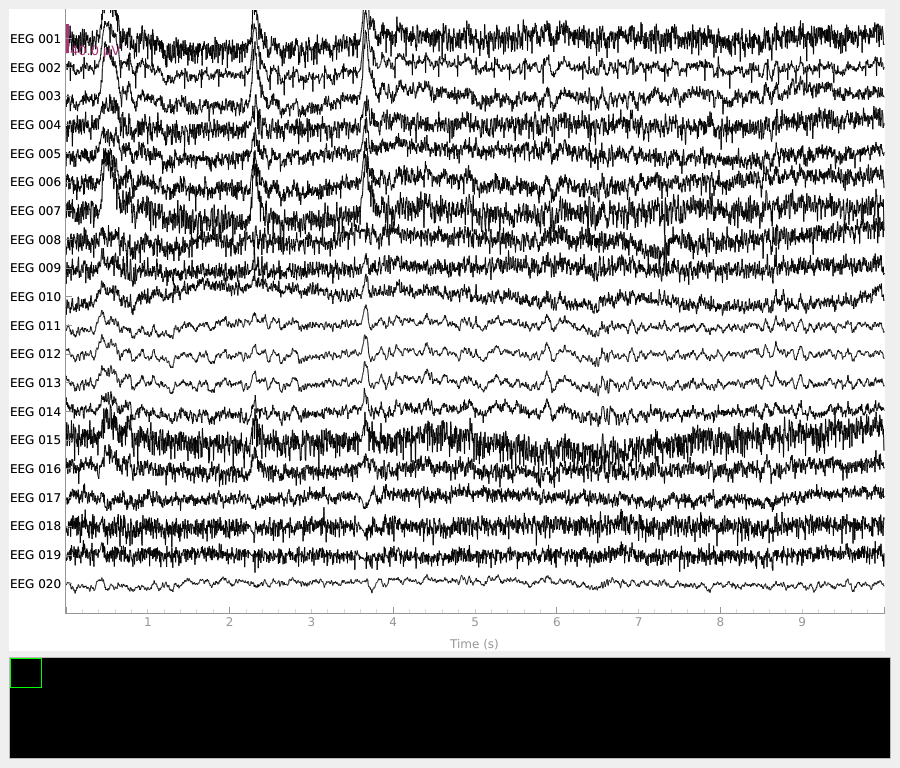•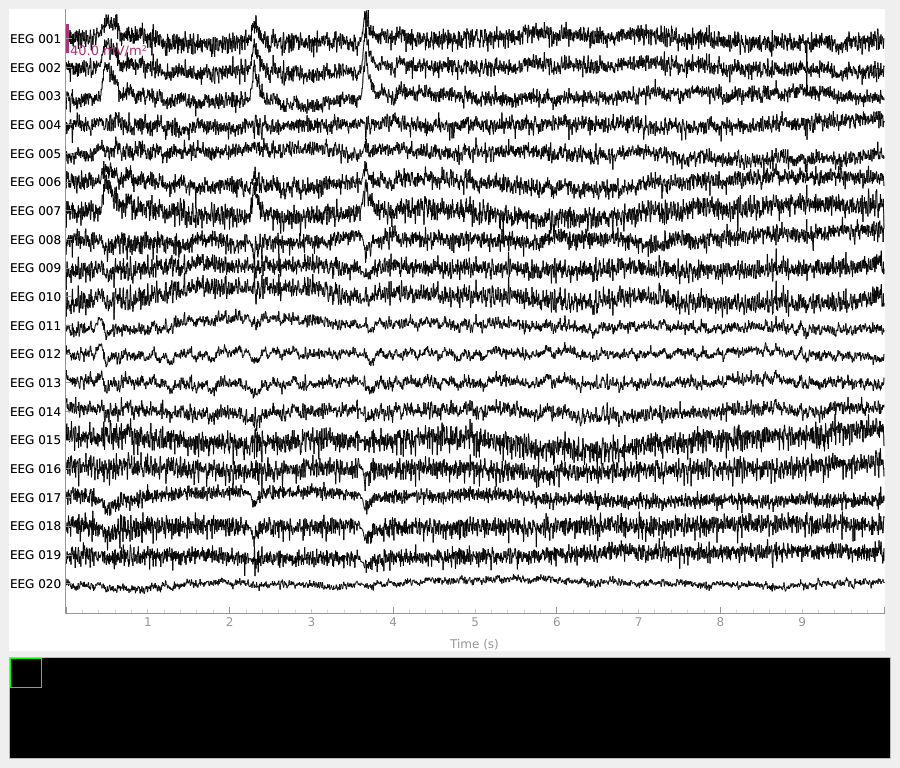```Fitted sphere radius:         91.0 mm
Origin head coordinates:      -4.1 16.0 51.7 mm
Origin device coordinates:    1.4 17.8 -10.3 mm
```

Also look at the power spectral densities:

```raw.plot_psd()
raw_csd.plot_psd()
```
•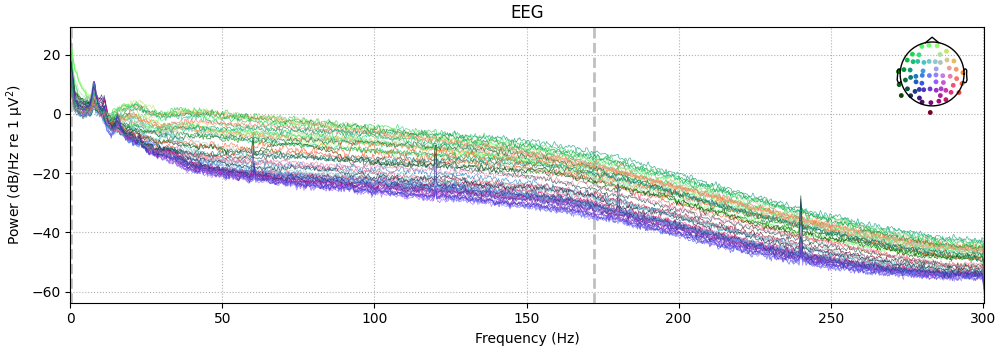•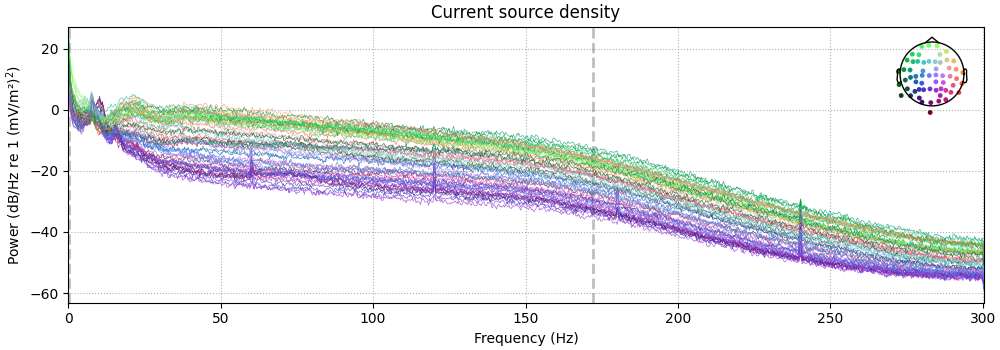```NOTE: plot_psd() is a legacy function. New code should use .compute_psd().plot().
Effective window size : 3.410 (s)
NOTE: plot_psd() is a legacy function. New code should use .compute_psd().plot().
Effective window size : 3.410 (s)
```

CSD can also be computed on Evoked (averaged) data. Here we epoch and average the data so we can demonstrate that.

```event_id = {'auditory/left': 1, 'auditory/right': 2, 'visual/left': 3,
'visual/right': 4, 'smiley': 5, 'button': 32}
epochs = mne.Epochs(raw, events, event_id=event_id, tmin=-0.2, tmax=.5,
evoked = epochs['auditory'].average()
```
```Not setting metadata
320 matching events found
Setting baseline interval to [-0.19979521315838786, 0.0] sec
Applying baseline correction (mode: mean)
Created an SSP operator (subspace dimension = 1)
1 projection items activated
Using data from preloaded Raw for 320 events and 421 original time points ...
```

First let’s look at how CSD affects scalp topography:

```times = np.array([-0.1, 0., 0.05, 0.1, 0.15])
evoked_csd = mne.preprocessing.compute_current_source_density(evoked)
evoked.plot_joint(title='Average Reference', show=False)
evoked_csd.plot_joint(title='Current Source Density')
```
•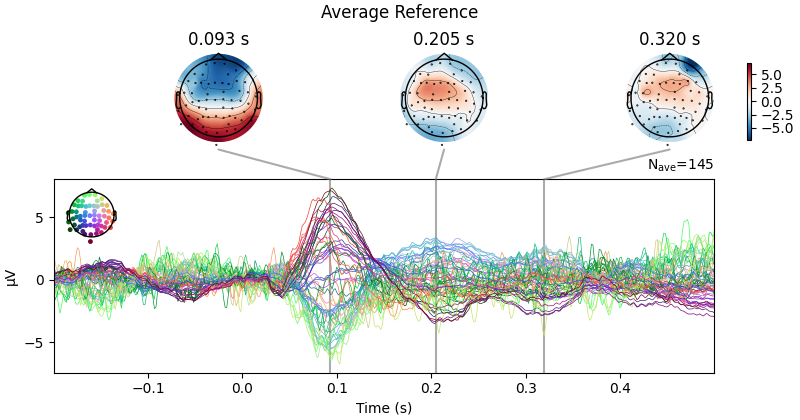•```Fitted sphere radius:         91.0 mm
Origin head coordinates:      -4.1 16.0 51.7 mm
Origin device coordinates:    1.4 17.8 -10.3 mm
Projections have already been applied. Setting proj attribute to True.
Projections have already been applied. Setting proj attribute to True.
```

CSD has parameters `stiffness` and `lambda2` affecting smoothing and spline flexibility, respectively. Let’s see how they affect the solution:

```fig, ax = plt.subplots(4, 4)
fig.set_size_inches(10, 10)
for i, lambda2 in enumerate([0, 1e-7, 1e-5, 1e-3]):
for j, m in enumerate([5, 4, 3, 2]):
this_evoked_csd = mne.preprocessing.compute_current_source_density(
evoked, stiffness=m, lambda2=lambda2)
this_evoked_csd.plot_topomap(
0.1, axes=ax[i, j], contours=4, time_unit='s',
colorbar=False, show=False)
ax[i, j].set_title('stiffness=%i\nλ²=%s' % (m, lambda2))
```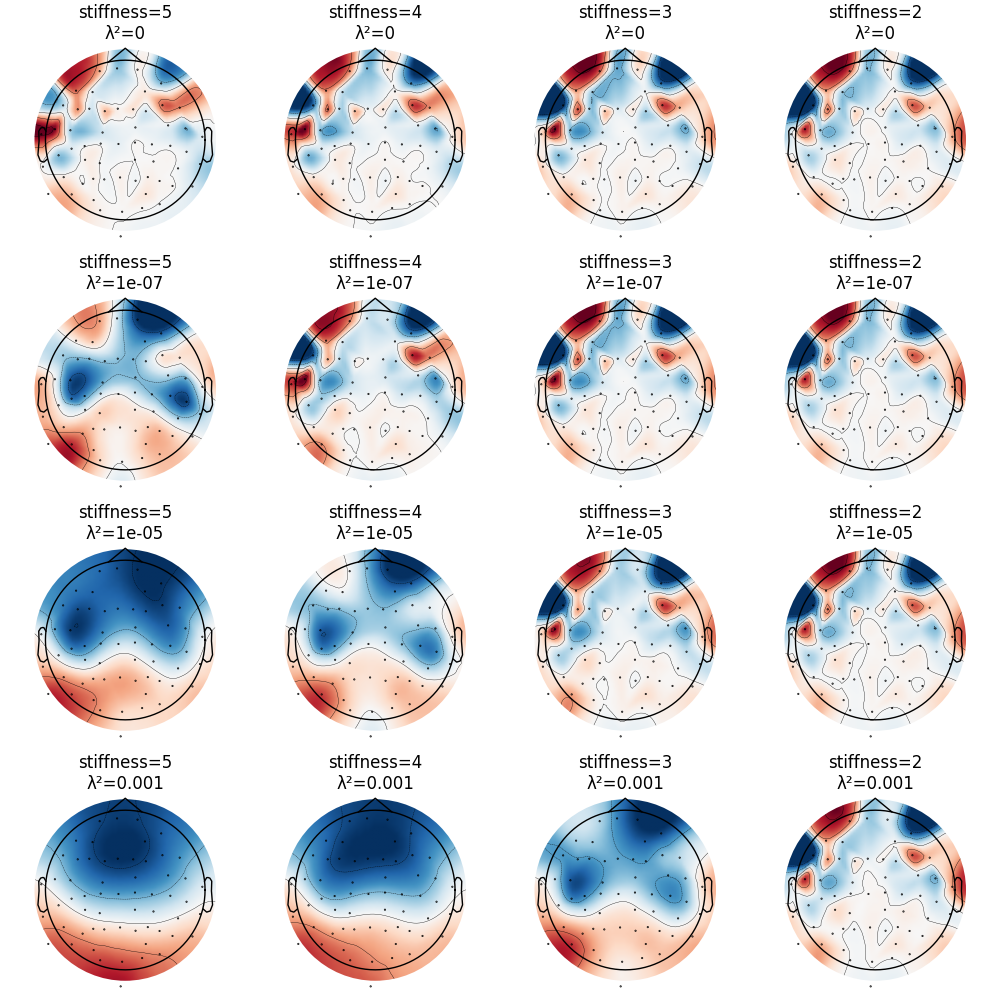```Fitted sphere radius:         91.0 mm
Origin head coordinates:      -4.1 16.0 51.7 mm
Origin device coordinates:    1.4 17.8 -10.3 mm
Origin head coordinates:      -4.1 16.0 51.7 mm
Origin device coordinates:    1.4 17.8 -10.3 mm
Origin head coordinates:      -4.1 16.0 51.7 mm
Origin device coordinates:    1.4 17.8 -10.3 mm
Origin head coordinates:      -4.1 16.0 51.7 mm
Origin device coordinates:    1.4 17.8 -10.3 mm
Origin head coordinates:      -4.1 16.0 51.7 mm
Origin device coordinates:    1.4 17.8 -10.3 mm
Origin head coordinates:      -4.1 16.0 51.7 mm
Origin device coordinates:    1.4 17.8 -10.3 mm
Origin head coordinates:      -4.1 16.0 51.7 mm
Origin device coordinates:    1.4 17.8 -10.3 mm
Origin head coordinates:      -4.1 16.0 51.7 mm
Origin device coordinates:    1.4 17.8 -10.3 mm
Origin head coordinates:      -4.1 16.0 51.7 mm
Origin device coordinates:    1.4 17.8 -10.3 mm
Origin head coordinates:      -4.1 16.0 51.7 mm
Origin device coordinates:    1.4 17.8 -10.3 mm
Origin head coordinates:      -4.1 16.0 51.7 mm
Origin device coordinates:    1.4 17.8 -10.3 mm
Origin head coordinates:      -4.1 16.0 51.7 mm
Origin device coordinates:    1.4 17.8 -10.3 mm